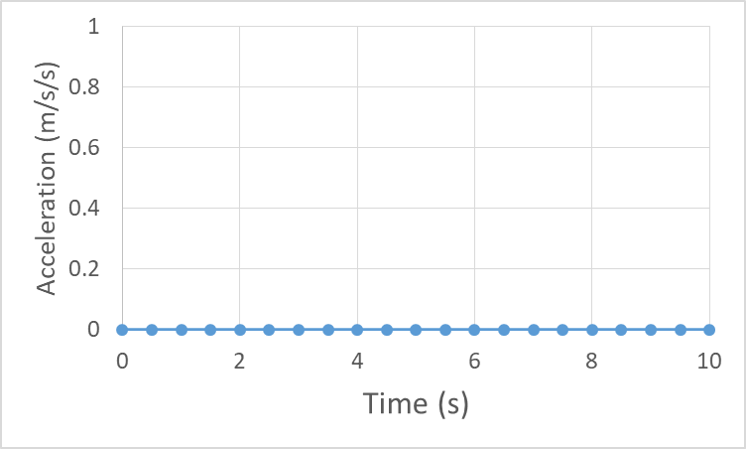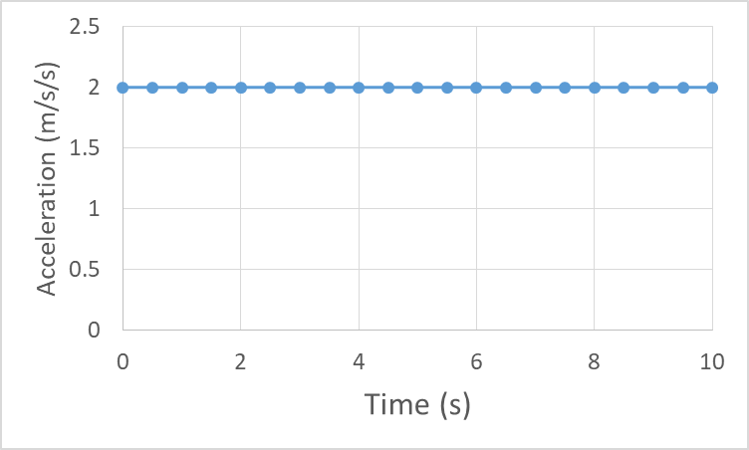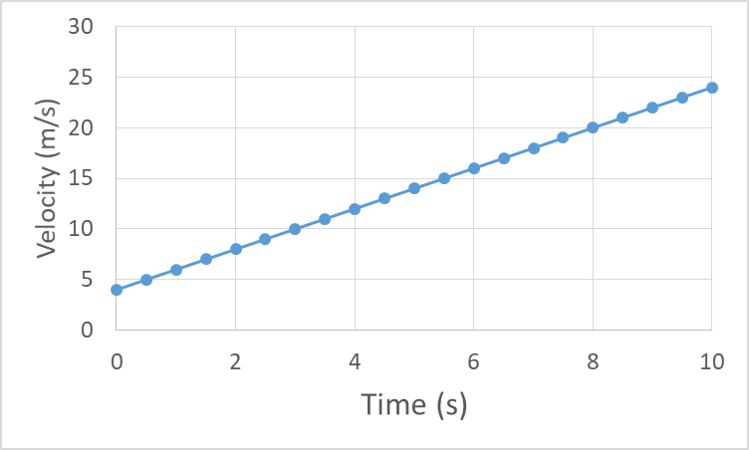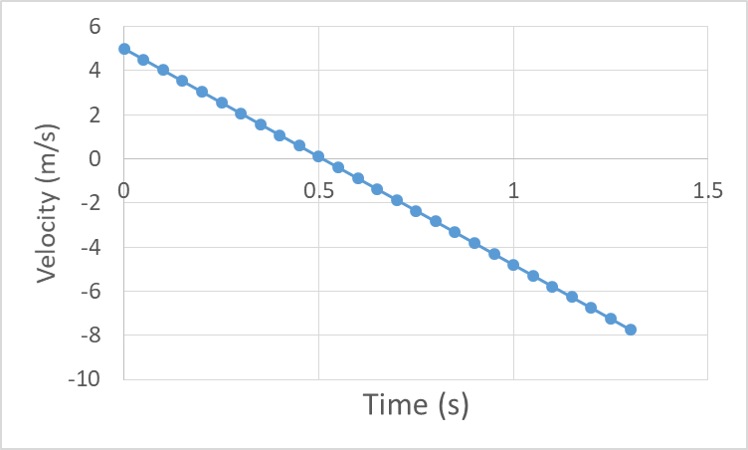# 8.7: Graphing Terminal Velocity and Other Motion

$$\newcommand{\vecs}{\overset { \rightharpoonup} {\mathbf{#1}} }$$ $$\newcommand{\vecd}{\overset{-\!-\!\rightharpoonup}{\vphantom{a}\smash {#1}}}$$$$\newcommand{\id}{\mathrm{id}}$$ $$\newcommand{\Span}{\mathrm{span}}$$ $$\newcommand{\kernel}{\mathrm{null}\,}$$ $$\newcommand{\range}{\mathrm{range}\,}$$ $$\newcommand{\RealPart}{\mathrm{Re}}$$ $$\newcommand{\ImaginaryPart}{\mathrm{Im}}$$ $$\newcommand{\Argument}{\mathrm{Arg}}$$ $$\newcommand{\norm}{\| #1 \|}$$ $$\newcommand{\inner}{\langle #1, #2 \rangle}$$ $$\newcommand{\Span}{\mathrm{span}}$$ $$\newcommand{\id}{\mathrm{id}}$$ $$\newcommand{\Span}{\mathrm{span}}$$ $$\newcommand{\kernel}{\mathrm{null}\,}$$ $$\newcommand{\range}{\mathrm{range}\,}$$ $$\newcommand{\RealPart}{\mathrm{Re}}$$ $$\newcommand{\ImaginaryPart}{\mathrm{Im}}$$ $$\newcommand{\Argument}{\mathrm{Arg}}$$ $$\newcommand{\norm}{\| #1 \|}$$ $$\newcommand{\inner}{\langle #1, #2 \rangle}$$ $$\newcommand{\Span}{\mathrm{span}}$$$$\newcommand{\AA}{\unicode[.8,0]{x212B}}$$

## Basic Motion Graphs

Motion graphs are a useful tool for visualizing and communicating information about an object’s motion. Our goal is to create motion graphs for our example skydiver, but first let’s make sure we get the basic idea.

We will start by looking at the motion graphs of on object with an initial position of 2 m and constant velocity of 4 m/s. An object moving at constant velocity has zero acceleration, so the graph of acceleration vs. time just remains at zero:The acceleration vs. time graph for an object with constant velocity is flat at zero.

The velocity is constant, so the graph of velocity vs. time will remain at the 4 m/s value:The velocity vs. time graph is flat (constant) at 4 m/s.

Velocity is the rate at which position changes, so the position v. time graph should change at a constant rate, starting from the initial position (in our example, 2 m). The slope of a motion graph tells us the rate of change of the variable on the vertical axis, so we can understand velocity as the slope of the position vs. time graph.The position vs. time graph is linear with a slope that is equal to the 4 m/s velocity and intercept that is equal to the 2 m initial position.

#### Reinforcement Exercises

What should be the value for the slope of the position vs. time graph of our example object? Calculate the slope of the position vs. time graph above and compare to your previous answer. [Hint: The initial position is 2 m and the position at a time of 2 s is 10 m. Slope is calculated as rise over run.]

Now let’s look at motion graphs for an object with constant acceleration. Let’s give our object the same initial position of 2 m, and initial velocity of 4 m/s, and now a constant acceleration of 2 m/s/s. The acceleration vs. time remains constant at 2 m/s/s:The acceleration vs. time graph is flat at the acceleration value, in this example 2 m/s/s

Acceleration is the rate at which velocity changes, so acceleration is the slope of the velocity vs. time graph. For our constant 2 m/s/s acceleration the velocity graph should have a constant slope of 2 m/s/s:The velocity vs. time graph is linear with a slope equal to the 2 m/s/s acceleration value and intercept equal to the initial velocity value of 4 m/s.

Finally, if the velocity is changing at a constant rate, then the slope of the position graph, which represents the velocity, must also be changing at a constant rate. The result of a changing slope is a curved graph, a curve with a constantly changing slope is a parabolic curve.The position vs. time graph of an object with constant acceleration is a parabolic curve. The curvature is upward for positive acceleration and downward for negative accelerations. The intercept is the initial position, in this example 2 m.

We haven’t made motion graphs for the situation of constant position because they are relatively unexciting. The position graph is constant at the initial value of position, the velocity graph is constant at zero and the acceleration graph is also constant at zero. Let’s end this section with some interesting graphs – those of an object that changes direction. For example, an object thrown into the air with an initial velocity of 5 m/s, from an initial position of 2 m that then falls to the ground at 0 m. Neglecting air resistance, the acceleration will be constant at negative g, or -9.8 m/s/s.The acceleration vs. time graph for an object is flat at -9.8 m/s/s (for a choice of downward as the negative direction).

The velocity will be positive, but slowing down toward zero, cross through zero as the object turns around, and then begin increasing in the negative direction.The velocity vs. time graph starts at 5 m/s and decreases linearly crossing through zero at roughly 0.5 s and then becoming more negative with time in linear fashion and reaching – 5 m/s just after 1 s. The slope is -9.8 m/s/s.

The position will increase as the object moves upward, then decrease as it falls back down, in a parabolic fashion because the slope is changing at a constant rate (acceleration is constant so velocity changes at a constant rate, so the slope of the position graph changes constantly).The position vs. time graph is a parabola with downward curvature starting at 2 m, peaking near 3.3 m at roughly 0.5 s, passing back through 2 m just after 1 s, and hitting the ground just after 1.3 s.

Check out this interactive simulation of a moving person and the associated motion graphs:

#### Everyday Example

Let’s look at the motion graphs for our skydiver while they are at a terminal velocity of -120 MPH, which is about 54 m/s. Let’s set our initial position for this analysis to be the position where they hit terminal velocity.

Acceleration is zero because they are at terminal velocity:Acceleration vs. time graph is constant (flat) at zero.

Velocity is constant, but negative:Velocity vs. time graph is constant near -52 m/s.

And position changes at a constant rate, becoming more negative with time.Position vs time graph decreases linearly from zero to -520 m after 10 s.

This page titled 8.7: Graphing Terminal Velocity and Other Motion is shared under a CC BY-NC-SA 4.0 license and was authored, remixed, and/or curated by Lawrence Davis (OpenOregon) via source content that was edited to the style and standards of the LibreTexts platform; a detailed edit history is available upon request.